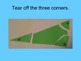Interior Angles of Triangles PowerPoint and FoldableSubject
Resource Type
Common Core Standards
Product Rating
File Type

Presentation (Powerpoint) File

8 MB|30 pages
Share
Product Description
The PowerPoint starts off reviewing types of angles. There is a foldable page you can have your students use to take notes on the angles if
you like.

Then there is an activity where the students work in a team to measure the three angles of the triangle with a protractor. The next
slide has the students tell you the three angles and add it up to (hopefully close to) 180. Then, it prompts them to remember that 180
is the same as straight line. The students are directed to tear off the corners and line them up to find a straight line.

My students loved this activity and always remembered the formula sum is 180 because of it.

Finally, we end by finding a missing angle of a triangle using
the formula for the interior angles of triangles:
Angle 1 + Angle 2 + Angle 3 = 180 degrees

Check out my Volume PowerPoint and Foldable.
Total Pages
30 pages